Design Library

# Improving an Air-Standard Power Cycle

## Our Purpose

We will examine several improvements that can be made to the simple air-standard cycle which increase its efficiency. We look at the addition of a regenerative heat exchanger, and then stages of reheating and intercooling.

## The basic Brayton cycle

In air-standard cycles, such as the Brayton cycle shown below in Figure 1, power is produced by a turbine expanding high temperature, high pressure air whose pressure has been achieved using a compressor. Unlike in liquid-vapor compression cycles such as a Rankine Cycle, the work needed to compress the working fluid in an air standard cycle is a large fraction of the work extracted by the turbine (often over 50%). The basic cycle's thermal efficiency hth is determined by the pressure ratio; the modified cycles' efficiencies depend upon the temperatures as well. Figure 1 shows the layout of a basic Brayton cycle.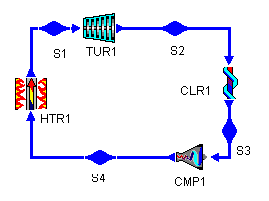Figure 1: a typical Brayton Cycle

We will start by constructing a typical Brayton cycle which we will use to judge the effects of our proposed changes. A Brayton cycle is the air-standard analog of the Rankine cycle. As such, its turbines and compressors are isentropic and adiabatic and its heaters, coolers, and heat exchangers are isobaric.

The example Brayton cycle (bray1.dsn)to which we will compare has the following parameters:

• turbine inlet pressure of 1 MPa
• turbine inlet temperature of 1000°C
• turbine exhaust pressure of 100 kPa
• turbine exhaust temperature of 15°C
and we note that its efficiencies with these operating parameters are
• Carnot efficiency: 77.37%
• thermal efficiency: 48.21%

We will compare the efficiencies of our modified Brayton cycles to these to see if the modifications have improved the cycle.

## Improving air standard cycle efficiencies

Improving cycle efficiency almost always involves making a cycle more like a Carnot cycle operating between the same high and low temperature limits. The Carnot cycle is maximally efficient because it receives all of its heat addition at the same temperature, which is the highest temperature in the cycle and rejects all of its heat at the lowest temperature in the cycle.

Figure 2 shows the T-s diagram for our example Brayton Cycle. We notice two things about this cycle. First, during the cooling process, we are throwing away quit a bit of heat. The second is that not all of the heat addition and rejection is at (or even near) the maximum and minimum cycle temperatures. We will look at each of these issues in turn.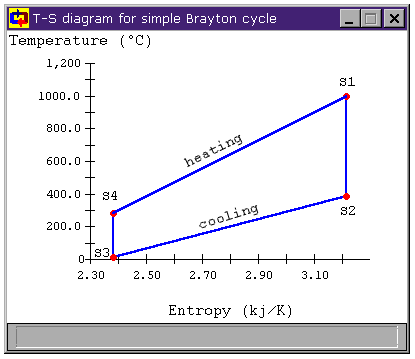Figure 2: Brayton Cycle T-s Diagram

Most cycles don't have all of their heat addition or rejection at one temperature. So, when we look to improve a cycle's efficiency, we often consider the mean temperature of heat addition, Ta and the mean temperature of heat rejection, Tr. These reflect what the temperature would have been if the same amount of heat had been added (or rejected) all at one temperature. They allow us to treat improving cycle efficiencies as we would for a Carnot cycle: by raising Ta or lowering Tr. For reversable heat transfer, the average temperature of heat addition is

Ta = Qin / DS

and the average temperature of heat rejection is

Tr = Qout / DS

Using these mean temperatures, we can find the cycle's thermal efficiency by applying the relation for Carnot efficiency to the mean cycle temperatures:

hth = (Ta - Tr) / Ta

Which will give same answer we get applying the usual hth = Wnet / Qhi relation.

For more efficient cycles, we would like to add heat at a higher temperature and reject it at a lower temperature. Obviously, if we could add all of our heat at the maximum cycle temperature and reject all of it at the minimum cycle temperature, our cycle's efficiency would equal the Carnot efficiency.

For instance, Table 1, shows these parameters in the simple Brayton cycle we have just considered.

 Table 1: Efficiency Parameters for simple Brayton Cycle Maximum Cycle Temperature 1000°C Mean Temperature of Heat Addition 592.7°C Minimum Cycle Temperature 15°C Mean Temperature of Heat Rejection 175.3°C Carnot Efficiency 77.37% Thermal Efficiency, hth 48.21%

We are adding heat at a much lower temperature (over 400°C lower) than the maximum cycle temperature. At the other end, we are removing heat from the system at 160°C above the minimum cycle temperature. We will now examine some modifications to the basic cycle and see if we cannot bring these numbers closer to the ideal.

## Improving Cycle Efficiency with a Regenerative Heat Exchanger

Could we use some of the heat rejected between states S2 and S3 to do some of the heating required between stages S4 and S1? The temperatures in the cycle are as follows:

 T1 = 1000°C T2 = 386°C T3 = 15°C T4 = 283°C

This seems to indicate that we can use the heat thrown out between S2 and S3 that is above 283°C to do some heating between S4 and S1. To take advantage of this potential heat, we add a heat exchanger to our cycle. Figure 3 shows the addition of such a heat exchanger (bray1x.dsn).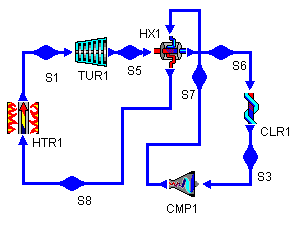Figure 3: Brayton Cycle with
Heat Exchanger

The heat exchanger shown in Figure 3 is assumed to be an ideal, counter-current one. For our purposes, this not only means that it is isobaric, but also that the cool side outlet can be heated up all the way to the temperature of the hot side inlet. So S8 = S5 and S6 = S7. Note that these are the maximum temperature differences we can achieve. With a less-than-ideal heat exchanger, we would not get quite such performance. (That is, S8 < S5 and S6 > S7.)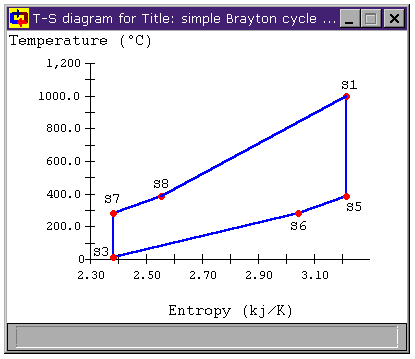Figure 4: Brayton Cycle with Heat Exchanger
T-s diagram

 Table 2: Brayton Cycle with Heat ExchangerEfficiency Parameters This Cycle SimpleBrayton Maximum Cycle Temperature 1000°C 1000°C Mean Temperature of Heat Addition 659.7°C 592.7°C Minimum Cycle Temperature 15°C 15°C Mean Temperature of Heat Rejection 134.5°C 175.3°C Carnot Efficiency 77.37% 77.37% Thermal Efficiency, hth 56.30% 48.21%

Examining the heating and cooling for the modified cycle in Figure 4, we can see that all of the heat transfer between S5 and S6 that was formerly thrown out in the cooler is now used to heat the fluid from state S7 and S8. Because of this, we are now only adding new heat to the cycle between S8 and S1, raising the mean temperature of heat addition. Similarly, we are now only removing heat from the cycle between S6 and S3, lowering the mean temperature of heat rejection. The result is that the cycle efficiency has risen substantially.

## Improving Cycle Efficiency with a Reheat Stage

To bring the average temperature at which we add heat to the cycle closer to the peak temperature, we can add a reheat stage to the cycle. Figure 5 shows the addition of such a stage to the standard Brayton cycle (bray1r.dsn). The intermediate pressure for the reheat stage is 300 kPa, giving each turbine roughly equal pressure ratios.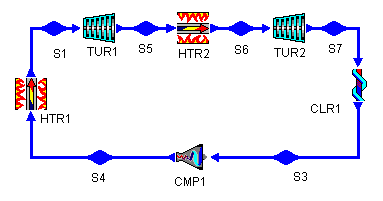Figure 5: Brayton Cycle with Reheat Stage

And, looking at Table 3 and the T-s diagram for this cycle shown in Figure 6, we can see that the average temperature of heat addition has increased. The original Brayton cycle is overlaid in light green in Figure 6 for comparison.Figure 6: Brayton Cycle with Reheat Stage
T-s diagram

 Table 3: Brayton Cycle with Reheat Efficiency Parameters This Cycle SimpleBrayton Maximum Cycle Temperature 1000°C 1000°C Mean Temperature of Heat Addition 654.7°C 592.7°C Minimum Cycle Temperature 15°C 15°C Mean Temperature of Heat Rejection 274.7°C 175.3°C Carnot Efficiency 77.37% 77.37% Thermal Efficiency, hth 40.96% 48.21%

However, the cycle efficiency of the modified cycle has actually decreased by over 7%. Why is this? Looking at Figure 6, we can see not only that the mean temperature of heat addition has increased, but also that the mean temperature of heat rejection has increased. So, while the the former increases efficiency, the latter decreases it. In this case, the net effect is a decrease in cycle efficiency (and a large decrease at that!).

## Improving Cycle Efficiency with Compressor Intercooling

In a similar fashion, we might look at Figure 2, an decide that we might improve the cycle by lowering the average temperature of heat rejection for the cycle.

To bring the average temperature at which we remove heat to the cycle closer to the lowest temperature, we can add an intercooling stage to the cycle. Figure 7 shows the addition of such a stage to a Brayton cycle (bray1i.dsn). Analogous to the reheat stage, the intermediate pressure for the intercooler is 300 kPa, giving each compressor roughly equal pressure ratios.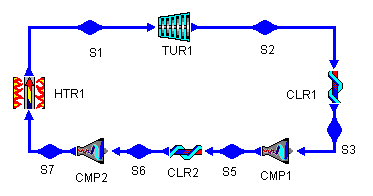Figure 7: Brayton Cycle with Intercooling

And, looking at the T-s diagram for this cycle shown in Figure 8, we can see that the average temperature of heat rejection has decreased. In the original Brayton cycle (overlaid in light green in Figure 8), the mean temperature of heat rejection was 175.3°C and the cycle shown in Figure 8 has a mean temperature of heat rejection of 145.0°C.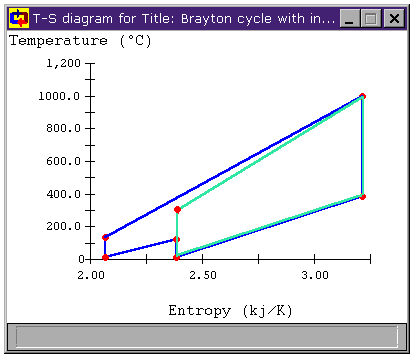Figure 8: Brayton Cycle with Intercooling
T-s diagram

 Table 4: Brayton Cycle with Intercooling Efficiency Parameters This Cycle SimpleBrayton Maximum Cycle Temperature 1000°C 1000°C Mean Temperature of Heat Addition 485.9°C 592.7°C Minimum Cycle Temperature 15°C 15°C Mean Temperature of Heat Rejection 145.0°C 175.3°C Carnot Efficiency 77.37% 77.37% Thermal Efficiency, hth 44.90% 48.21%

However, as we saw with the addition of a reheat stage, the cycle efficiency of the modified cycle has once again decreased. Again, though we have met our objective of decreasing the mean temperature of heat rejection, it is also clear that the mean temperature of heat addition has decreased as well, having a negative impact on cycle efficiency.

## Combining Regeneration with Compressor Intercooling and Turbine Reheat

In the last three sections, we considered the effect of additional components on the efficiency of the Brayton cycle. We added a regenerative heat exchanger, a turbine reheat stage, and a compressor intercooling stage. However, we saw that only the regenerative heat exchanger had a positive effect on cycle efficiency.

The reheat and intercooling stages have their places, though. When we added a heat exchanger to the Brayton cycle, we took advantage of waste heat being thrown away in the cooler and used it to provide heat that would be used in the turbine. However, we were limited by the temperature difference between the air leaving the turbine and the air leaving the compressor. For our example, that temperature difference was about 100°C. The larger that temperature difference, the more heat we can grab from the cooling process and inject into the air before it enters the heater and the greater portion of our added heat is near the maximum cycle temperature.

Can we make that temperature difference greater? When we look at the T-s diagrams in Figure 6 and Figure 8, we see that the difference in temperature between the air leaving the compressor and the air leaving the turbine in either cycle is larger than it was for the unmodified Brayton cycle in both cases. Below, Figure 9 shows the T-s diagram for a cycle with both intercooling and reheating (bray1ri.dsn). For reference, the unmodified Brayton cycle's T-s diagram is shown in light green. Also shown is the temperature difference for the unmodified Brayton cycle DT1 and the temperature difference of the cycle with both intercooling and reheat stages DT2. We can see that the latter is much greater. In fact, whereas DT1 is about 100°C, DT2 is over 500°C!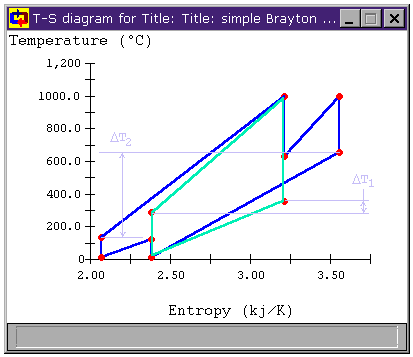Figure 9: Brayton Cycle with both
Reheat and Intercooling T-s diagram

 Table 5: Brayton Cycle with Reheat and Intercooling Efficiency Parameters This Cycle SimpleBrayton Maximum Cycle Temperature 1000°C 1000°C Mean Temperature of Heat Addition 559.6°C 592.7°C Minimum Cycle Temperature 15°C 15°C Mean Temperature of Heat Rejection 230.4°C 175.3°C Carnot Efficiency 77.37% 77.37% Thermal Efficiency, hth 39.52% 48.21%

We note that the thermal efficiency of this cycle is still worse than that for the basic Brayton cycle (in fact, it's worse than for the cycles with only reheat or intercooling as well). However, we can take advantage of the larger temperature difference with a heat exchanger. This new cycle (bray1rix.dsn) is shown below in Figure 10.Figure 10: Brayton Cycle with Reheat, Intercooling,
and Regererative Heat Exchange

The T-s diagram and efficiency parameters for this new cycle is shown below. We note that the cycle's thermal efficiency is now 20% better than that of the basic Brayton cycle and within 10% of the Carnot efficiency, which is very impressive.Figure 11: Brayton Cycle with Regeneration,
Reheat, and Intercooling T-s diagram

 Table 6: Brayton Cycle with Regeneration, Reheat, and Intercooling Efficiency Parameters This Cycle SimpleBrayton Maximum Cycle Temperature 1000°C 1000°C Mean Temperature of Heat Addition 811.3°C 592.7°C Minimum Cycle Temperature 15°C 15°C Mean Temperature of Heat Rejection 68.2°C 175.3°C Carnot Efficiency 77.37% 77.37% Thermal Efficiency, hth 68.51% 48.21%

## Additional Stages of Regeneration, Reheat, and Intercooling

The last section investigated the addition of a single stage of regenerative heat exchange, reheating, and intercooling to the basic Brayton cycle and revealed a substantial improvement in the cycle's thermal effciency. It seems natural to ask how cycle efficiency would be improved with even more stages. Figure 12 below shows the layout of such a cycle with 5 turbines and 5 compressors (bray5rix.dsn). The pressures are chosen to give each turbine and compressor roughly the same pressure ratio as the others.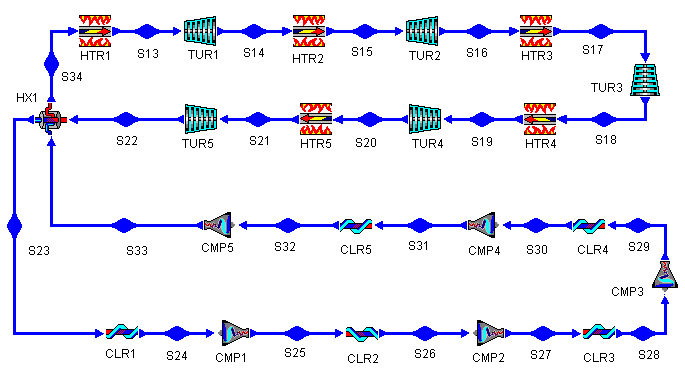Figure 12: Brayton Cycle with Regeneration, Reheat, and Intercooling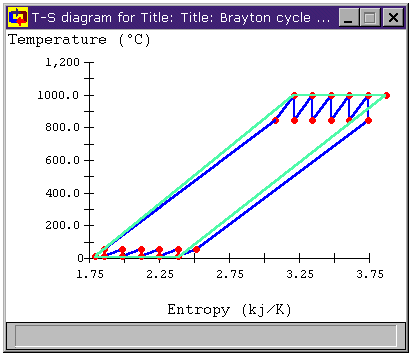Figure 13: Brayton Cycle with Multiple Stages of
Regeneration, Reheat, and Intercooling T-s diagram

Additionally, we can see from Figure 13, that the cycle is approaching the ideal Carnot cycle, with all of the heat addition near the maximum cycle temperature and all of the heat rejection near the minimum cycle temperature. For comparison, the T-s diagram of the Carnot cycle is overlaid in light green.

 Table 7: Brayton Cycle with Multiple Regeneration,Reheat, and Intercooling Stages Efficiency Parameters Number of Turbines SimpleBrayton 1 2 3 4 5 Maximum Cycle Temperature 1000°C Mean Temperature of Heat Addition 592.7°C 659.7°C 811.8°C 870.1°C 900.9°C 920.0°C Minimum Cycle Temperature 15°C Mean Temperature of Heat Rejection 275.3°C 134.5°C 68.0°C 49.0°C 40.2°C 34.8°C Carnot Efficiency 77.37% Thermal Efficiency, hth 48.2% 56.3% 68.6% 71.8% 73.3% 74.2%

## Choosing Intermediate Pressures

In each Brayton cycle with more than one turbine, we have chosen the pressures so that the pressure ratio (PR) for each turbine is roughly the same as for all the rest of them. The same is true for the compressors. Why do we take this approach?

There are two reasons. First, in keeping with our overall goal of improving cycle efficiency, we want the mean temperature of heat addition to be as high as possible and the mean temperature of heat rejection to be as low as possible. It turns out (and this can be shown with a little math) that we achieve this by keeping the PRs of the turbines even.

The other reason is a purely pragmatic design consideration. If we are going to order turbines, larger ones tend to be more expensive than smaller ones (both in initial cost and in overhead for physical space and maintenance). The same will tend to be true of compressors.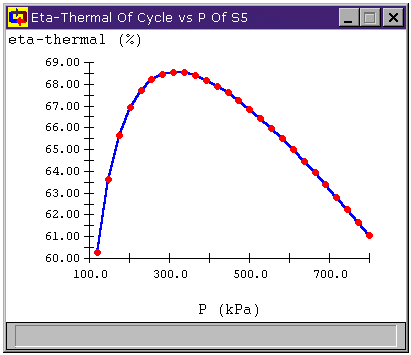Figure 14: Cycle efficiency vs. turbine outlet pressure

The overall PR for the cycle is 1000 kPa / 100 kPa = 10. For each turbine to have the same PR, each PRturbine must be (PRoverall)1/(#turbines).

• Download the CyclePad design of the Brayton cycle with a regenerative heat exchanger, intercooling, and reheat bray1rix.dsn.
• Download the CyclePad design of the 5 turbine Brayton cycle with a regenerative heat exchanger, intercooling, and reheat bray5rix.dsn.

## Sources

Haywood, R.W. 1980. Analysis of Engineering Cycles. Pergamon Press. ISBN: 0-08-025440-3

Van Wylen, Sonntag, Borgnakke. 1994. Fundamentals of Classical Thermodynamics, 4th Ed. John Wiley and Sons. ISBN: 0-471-59395-8

Contributed by: M. E. Brokowski
Initial Entry: 02/02/1998
Last Edited: 03/04/1998